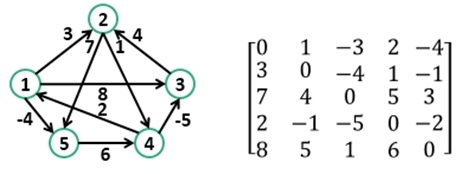# Floyd Warshall Algorithm

Floyd-Warshall algorithm is used to find all pair shortest path problem from a given weighted graph. As a result of this algorithm, it will generate a matrix, which will represent the minimum distance from any node to all other nodes in the graph.At first, the output matrix is the same as the given cost matrix of the graph. After that, the output matrix will be updated with all vertices k as the intermediate vertex.

The time complexity of this algorithm is O(V^3), where V is the number of vertices in the graph.

## Input and Output

Input: The cost matrix of the graph.
0 3 6 ∞ ∞ ∞ ∞
3 0 2 1 ∞ ∞ ∞
6 2 0 1 4 2 ∞
∞ 1 1 0 2 ∞ 4
∞ ∞ 4 2 0 2 1
∞ ∞ 2 ∞ 2 0 1
∞ ∞ ∞ 4 1 1 0

Output:
Matrix of all pair shortest path.
0 3 4 5 6 7 7
3 0 2 1 3 4 4
4 2 0 1 3 2 3
5 1 1 0 2 3 3
6 3 3 2 0 2 1
7 4 2 3 2 0 1
7 4 3 3 1 1 0

## Algorithm

floydWarshal(cost)

Input − The cost matrix of given Graph.

Output: Matrix to for shortest path between any vertex to any vertex.

Begin
for k := 0 to n, do
for i := 0 to n, do
for j := 0 to n, do
if cost[i,k] + cost[k,j] < cost[i,j], then
cost[i,j] := cost[i,k] + cost[k,j]
done
done
done
display the current cost matrix
End

## Example

#include<iostream>
#include<iomanip>
#define NODE 7
#define INF 999
using namespace std;

//Cost matrix of the graph
int costMat[NODE][NODE] = {
{0, 3, 6, INF, INF, INF, INF},
{3, 0, 2, 1, INF, INF, INF},
{6, 2, 0, 1, 4, 2, INF},
{INF, 1, 1, 0, 2, INF, 4},
{INF, INF, 4, 2, 0, 2, 1},
{INF, INF, 2, INF, 2, 0, 1},
{INF, INF, INF, 4, 1, 1, 0}
};

void floydWarshal() {
int cost[NODE][NODE];    //defind to store shortest distance from any node to any node
for(int i = 0; i<NODE; i++)
for(int j = 0; j<NODE; j++)
cost[i][j] = costMat[i][j];     //copy costMatrix to new matrix

for(int k = 0; k<NODE; k++) {
for(int i = 0; i<NODE; i++)
for(int j = 0; j<NODE; j++)
if(cost[i][k]+cost[k][j] < cost[i][j])
cost[i][j] = cost[i][k]+cost[k][j];
}

cout << "The matrix:" << endl;
for(int i = 0; i<NODE; i++) {
for(int j = 0; j<NODE; j++)
cout << setw(3) << cost[i][j];
cout << endl;
}
}

int main() {
floydWarshal();
}

## Output

The matrix:
0  3  5  4  6  7  7
3  0  2  1  3  4  4
5  2  0  1  3  2  3
4  1  1  0  2  3  3
6  3  3  2  0  2  1
7  4  2  3  2  0  1
7  4  3  3  1  1  0